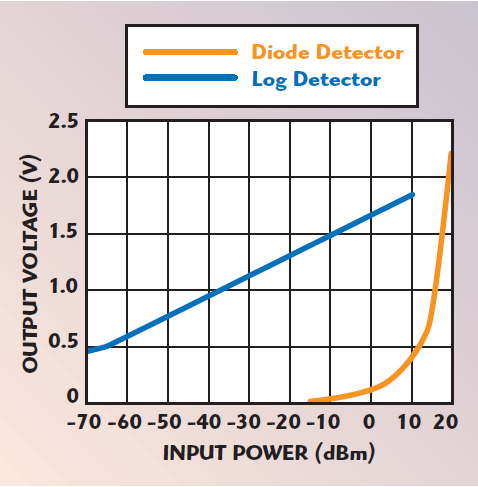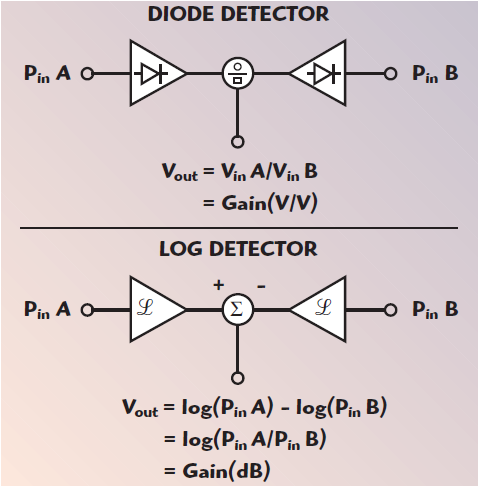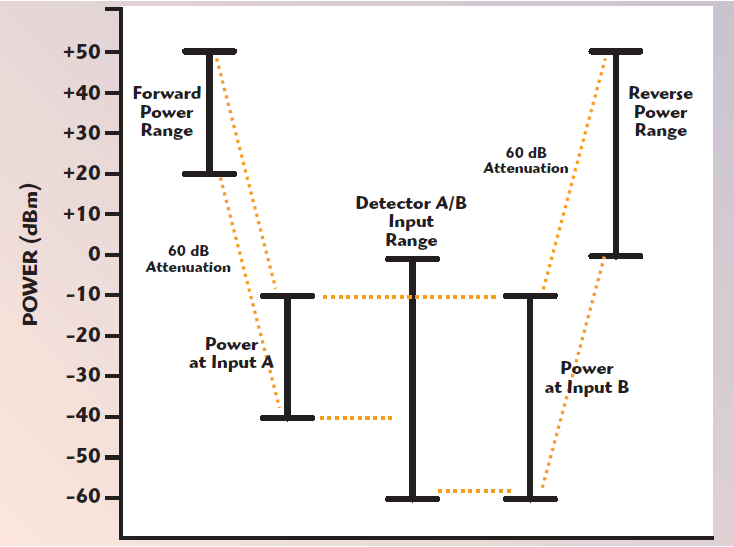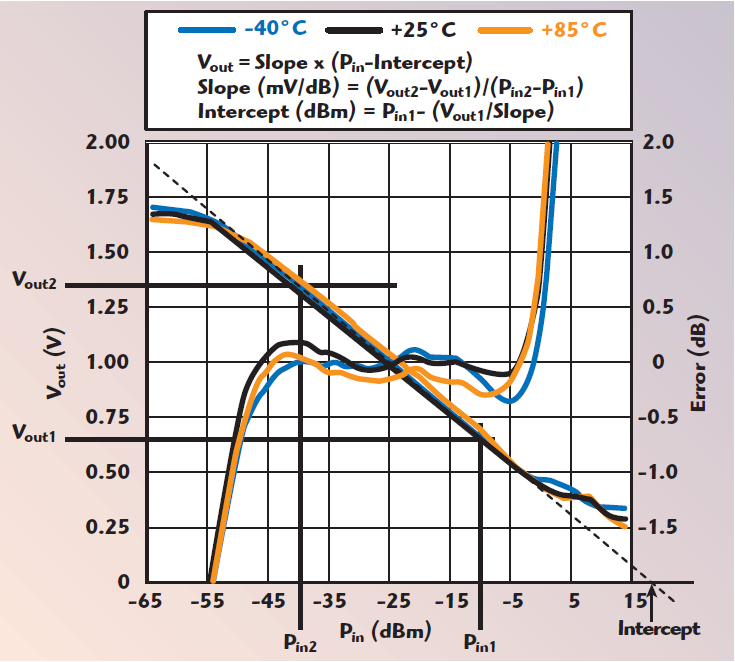# Measuring VSWR and Gain in Wireless Systems

Measurement and control of gain and reflected power in wireless transmitters are critical auxiliary functions that are often overlooked. The power reflected back from an antenna is specified using either the voltage standing wave ratio (VSWR) or the reflection coefficient (also referred to as return loss). Poor VSWR can cause shadowing in a TV broadcast system as the signal reflected off the antenna reflects again off the power amplifier and is then rebroadcast. In wireless communications systems, shadowing will produce multi-path-like phenomena. While poor VSWR can degrade transmission quality, the catastrophic VSWR that results from damage to coaxial cable or to an antenna can, at its worst, destroy the transmitter. The gain of a signal chain is measured and controlled as part of the overall effort to regulate the transmitted power level. If too much or too little power is transmitted, the result will be either violation of emissions regulations or a poor quality link. The reflection coefficient is calculated by measuring the ratio between forward and reverse power. Gain, on the other hand, is calculated by measuring input and output power. The high commonality of hardware used to measure gain and VSWR can reduce overall component count. This article will focus on techniques that can be used to perform these in-situ measurements in wireless transmitters.

### A Typical Wireless Transmitter

Figure 1 shows a typical wireless transmitter. It consists of mixed-signal base band circuitry, an up-converter (which generally includes one or more intermediate frequencies or IFs), amplifiers, filters and a power amplifier. These components may be located on different PCBs or may even be physically separated. In the example shown, an indoor unit is connected to an outdoor unit with a cable. In such a configuration, both units may be expected to have well defined, temperature-stable gains. Alternatively, each unit might be expected to deliver a well-defined output power. There are two different approaches to the ultimate goal of delivering a known power level to the antenna: power control or gain control.Figure 1. Power control versus gain control.

With power control, the system relies on being able to precisely measure the output power (using detector D in this example). Once the output power has been measured, the gain of some component in the system (in this case, it might be the IF VGA) is varied until the correct output power is measured at the antenna. It is not necessary to know the gain of the circuit or the exact input signal amplitude; it is just a matter of varying the gain or input signal until the output power is correct. This approach is often (incorrectly) referred to as automatic gain control or AGC. To be correct, it should be referred to as automatic power control or APC since it is power not gain that is being precisely regulated.

Gain control takes a different approach. Here, at least two power detectors are used to precisely regulate the gain of the complete signal chain or a part thereof. A precise input signal is then applied to the signal chain. A number of factors ultimately determine which approach is used. Power control requires only one power detector and makes sense in a non-configurable transmitter whose components are fixed. For example, power could be measured at the output of the RF HPA but adjustments would be made using the IF VGA. Gain control, on the other hand, may make more sense in a reconfigurable system whose components come from different vendors. In the example, the input power and output power of the HPA are being measured (using detectors C and D) so the gain can be regulated independent of the other blocks in the circuit. Note that the power/gain control loops can be all analog or microprocessor based. Gain control would be less practical in the example since the two required detector signals (detectors A and D) are physically remote from one another. A more practical approach would be to independently control the gain of the indoor and outdoor units.

### RF Detectors

Until recently, most RF power detectors were built using a temperature-compensated half-wave rectifying diode circuit. These devices deliver an output voltage that is proportional to the input voltage over a limited dynamic range (typically 20 to 30 dB). As a result, the relationship between output voltage and input power in dBm is exponential (see Figure 2). While the temperature stability of a temperature-compensated diode detector is excellent at high input powers (+10 to +15 dBm), it degrades significantly as the input drive is reduced. A log detector, on the other hand, delivers an output voltage proportional to the log of the input signal over a large dynamic range (up to 100 dB). The temperature stability is usually constant over the complete dynamic range. A log-responding device offers a key advantage in gain and VSWR measurement applications. In order to compute the gain or the reflection loss, the ratio of the two signal powers (either OUTPUT/INPUT or REVERSE/FORWARD) must be calculated (see Figure 3). An analog divider must be used to perform this calculation with a linear-responding diode detector, but only simple subtraction is required when using a log-responding detector (since log (A/B) = log (A) – log (B)). A dual RF detector has an additional advantage compared to a discrete implementation. There is a natural tendency for two devices (RF detectors in this case) to behave similarly when they are fabricated on the same silicon wafer. Both devices will have similar temperature drift characteristics, for example. At the summing node, this drift will cancel to yield a more temperature-stable result.Figure 2. Transfer functions of diode and log detectors.Figure 3. Calculating the gain using diode and log detectors.

### Gain Measurement Example

Figure 4 shows a transmitter whose gain is regulated using a dual power detector. The simplified transmit signal chain shown consists of a high performance IF-synthesizing DAC, a VGA, a mixer/upconverter and a high power amplifier. High performance DACs, such as the AD9786 and AD9779 that run at sampling frequencies up to 500 MSPS and beyond, are capable of synthesizing intermediate frequency outputs (100 MHz in this example). The output of the DAC is Nyquist filtered using a bandpass filter before being applied to an ADL5330 variable gain amplifier. Conveniently, the amplifier accepts a differential input that can be tied directly to the output of the differential filter. This, in turn, is tied to the DAC output. The VGA output is converted from differential to single-ended using a balun transformer, and is then applied to the ADL5350 mixer. After appropriate filtering (not shown), the signal is amplified and transmitted at a maximum output power level of 30 W (approximately +45 dBm).Figure 4. Gain control using a dual rms-responding log detector.

The gain of the signal chain is measured by detecting the power at the DAC output and at the output of the HPA. The gain is then regulated by adjusting the gain of a VGA. At the DAC and PA outputs, a sample of the signal is taken and fed to the detectors. At the HPA output, a directional coupler is used to tap off some of the power going to the antenna. The transfer function of the AD8364 dual detector (see Figure 5) shows that at the output frequency used (2140 MHz in this case), the detector has the best linearity and the most stable temperature drift at power levels below –10 dBm. Thus, the power coming from the directional coupler (+25 dBm max) must be attenuated before being applied to the detector. If maximizing the detector dynamic range is not critical to the application, the attenuation can be conservatively set at 41 dB so that the detector sees a maximum input power of –16 dBm. This still leaves about 34 dB of useful dynamic range over which the gain can be controlled. To detect the input power level at the DAC output, a directional coupler is impractical at this low frequency. In addition, directional coupling is not necessary since there will be little or no reflected signal at this point in the circuit. Furthermore, the power being delivered to the VGA is –10 dBm, so the power to be delivered to the detector is only 6 dB lower. Since the detector has an input impedance of 200 Ω and the VGA has an input impedance of 50 Ω, it quickly becomes clear that the two devices can simply be connected in parallel. With the same voltage present at both inputs, the 50 to 200 Ω impedance ratio will result in a convenient 6 dB power difference. Where high measurement precision is required, care must be paid to the temperature stability of the power detectors. This issue is further complicated if the temperature drift characteristics of the detectors change with frequency. The dual detector shown provides temperature compensation nodes. The temperature compensation is activated by connecting a voltage to the ADJ pins of each detector (this voltage can be conveniently derived using a resistor divider from the 2.5 V on-chip reference). No compensation is required for the low frequency input (ADJB is grounded), while a 1 V compensation voltage is required at ADJA to minimize temperature drift at 2.1 GHz. While the focus of the application circuit is gain measurement, it should be noted that input power and output power can also be measured. The outputs of the individual detectors are available and can be separately sampled. Because the detectors are log responding, their outputs can be simply subtracted to yield gain. This subtraction is performed on chip and the gain result is delivered as a differential voltage. The full-scale differential voltage is approximately ±4 V (biased up to 2.5 V) with a slope of 100 mV/dB. Digitizing with a 10-bit ADC with an LSB size of ~10 mV (±5 V full scale), 0.1 dB measurement resolution is achievable.Figure 5. Gain transfer function of a dual rms-responding log detector.

### VSWR Measurement Example

A dual log detector can also be used to measure the reflection coefficient of an antenna. In Figure 6, two directional couplers are used, one to measure the forward power and one to measure the reverse power. As in the previous example, additional attenuation is required before applying these signals to the detectors. The AD8302 dual detector has a measurement range of ±30 dB. The level planning used in this example is graphically depicted in Figure 7. In this example, the expected output power range from the HPA is 30 dB, from +20 to +50 dBm. Over this power range, reflection coefficients from 0 dB (short or open load) up to –20 dB should be able to be accurately measured. Each of the AD8302’s detectors has a nominal input range from 0 to –60 dBm. In this example, the maximum forward power of +50 dBm is padded down to –10 dBm at the detector input. When the HPA is transmitting at its lowest power level of +20 dBm, the detector sees a power of –40 dBm, still well within its input range.Figure 6. Return loss measurement using a dual log detector.Figure 7. Level planning for VSWR measurement using a dual log detector.

The power from the reverse path is padded down by the same amount. This means that the system is capable of measuring reflected power up to 0 dB. This may not be necessary if the system is designed to shut down when the reflection coefficient degrades below a certain minimum (such as 10 dB), but it is permissible because the detector has so much dynamic range. For example, when the HPA is transmitting +20 dBm, the reverse path detector will see an input power of –60 dBm if the antenna has a return loss of 20 dB. The application circuit provides a direct reading of return loss, but no information is provided about the absolute forward or reverse power. If this information is required, the dual detector used in the gain control would be more useful because it would provide a measure of absolute forward and reflected power along with the reflection coefficient. The dual log detector used in the return loss measurement also provides a phase output. Because of the large gain in the main signal path of a progressive compression log amp, a limited (amplitude saturated) version of the input signal is a natural by product. These limiter outputs are multiplied together to yield a phase-detected output with a range of 180° centered around an ideal operating point of 90°. In a VSWR application, this information constitutes the phase angle of the reflected signal (with respect to the incident signal) and may be of use in optimizing the power delivered to the antenna.

### Amplifier Gain Measurement Using A Single Log Detector And An RF Switch

Figure 8 shows an alternative approach to gain measurement, which is also applicable to VSWR measurement. In this application, measuring and controlling the gain of a PA is desired. The PA in the example is running at 8 GHz and has an output power range from +20 to +50 dBm. This is a fixed-gain PA, so the output power is adjusted by changing input power. Two directional couplers are used to detect input and output power. However, there is only a single log detector so the two signals are alternately connected to the detector using a single-pole, double-throw RF switch. The AD8317 detector has a 0 to –50 dBm input range at this frequency. To measure the gain, the input and output powers are alternately measured and digitized. The results are then simply subtracted to yield gain. Once the gain is known, the digital control loop is completed by making any necessary adjustments to the gain of the PA via a bias adjustment. The level planning for this example is shown in Figure 9. Attenuation is used so that the two input power levels at the RF switch are close together and within the input range of the detector.Figure 8. Gain measurement using a single log detector.Figure 9. Level planning for gain measurement using a single log detector.

### Precise Gain Measurement Without Factory Calibration

In addition to reducing component count, this gain measurement method has a number of interesting features. Because the same circuit is being used to measure input and output power, it is possible to make precise, temperature-stable gain measurements without ever calibrating the circuit. A look at the nominal transfer function of a log detector will help in understanding why (see Figure 10).

To figure out the unknown, PIN, the equation can be rewritten as

Since gain is the difference in the measured input powers (the different attenuation levels of the two paths still have to be factored in), it can be written as

Therefore, the intercept of the detector is not required to calculate the gain. Even though the slope of a detector will change from device to device and over temperature, if VOUT1 and VOUT2 are close to each other (it can be done with good level planning and because of the finite input range of the detector), a typical value for the slope can be taken directly from the datasheet and used in the above calculation.Figure 10. Calibrating a log detector.

### Output Power Monitoring

In the gain measurement using a single log detector, the power is measured in order to calculate gain, so the system shown can also be used to monitor the output power. However, this cannot be done precisely without factory calibration. To calibrate the circuit, the antenna must be temporarily replaced by a power meter. The output power and detector voltages are then measured at two points within the linear range of the detector. These numbers would then be used to calculate the slope and intercept of the detector. For optimum precision, the detector includes a temperature compensation pin. A resistor is connected between this pin and ground to reduce the temperature drift to approximately ±0.5 dB at the frequency of operation (8 GHz in the example shown). As a result, it is not necessary to do any additional calibration over temperature.

### Conclusion

Because of their linear-in-dB transfer function, log amplifiers can be easily used to measure gain and return loss. When dual devices are used, very high measurement precision is achievable. In some cases, this can be achieved without factory calibration. In all cases, careful power level planning is necessary so that the power detectors are driven at power levels that offer good linearity and temperature stability.